Q.1
Two situations are shown shown below of a dice. If there is 4 in the bottom then what will be on the top ?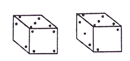Q.2
Two situations are shown shown below of a dice. Which number is in front of 5 ?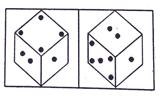Q.3
Two parts is shown below of a dice. If the number 'four' is above then which number will be in the bottom ?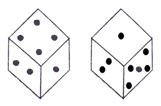Q.4
Two situations are shown  below of a dice. If there is 4 on the top, then what will be the number of bottom ?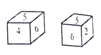Q.5
What is the point in opposite side of pane which has three points of the dice ?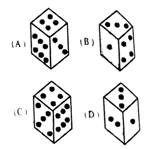Q.6
What is the point on pane in front of pane which has two points of the dice ?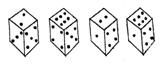Q.7
For the following question first understand vane of dice and then answer the question. Which is the number in front of vane 6 ?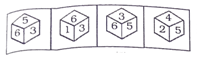Q.8
Understand the dice faces first and then answer the question ? Which is the color in front of red vane ?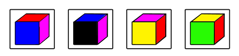Q.9
In the following picture four form are shown of a dice. Which is the number on vane in front of 3 ?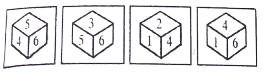Q.10
Four Conditions of the dice is shown below : When 6 is on top then what will be the number in the bottom ?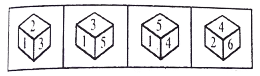Q.11
Three condition are shown of a dice. Then tell about the part of the other side 's about three points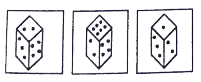Q.12
Four placement situations are shown below in the following figures. If the top number is 6, then find the number of the bottom ?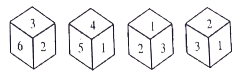Q.13
Consider the following two known shapes of the dice which is the number in front of 5 ?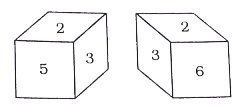Q.14
Three installation situations are shown below of a dice. Find the number of surface which is opposite of surface number 6 ?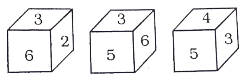Q.15
What is the number on the vane in behind of the vane of number 4 ?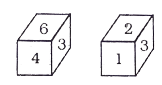Q.16
Four situations are given of a dice. Identify that if 5 is on the top then what number will be on the bottom ?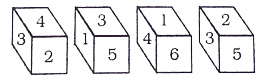Q.17
Four times a dice is thrown and four different shapes are shown below. Find the number on the vane in front of that vane which show 2 ?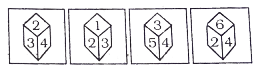Q.18
Which is the number in front of 2 in the following two different shapes of a dice ?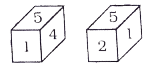Q.19
On the basis diagram which letter is the letter on the opposite surface of A ?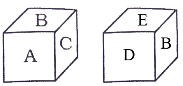Q.20
There is a  six vane of a dice. A, B, C, D, E and F. A is near to B. B is close to D but not to near C. E is near to D and F. which is the vane in front of A ?

Scroll